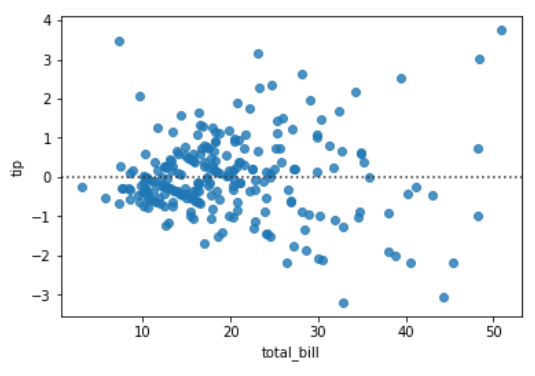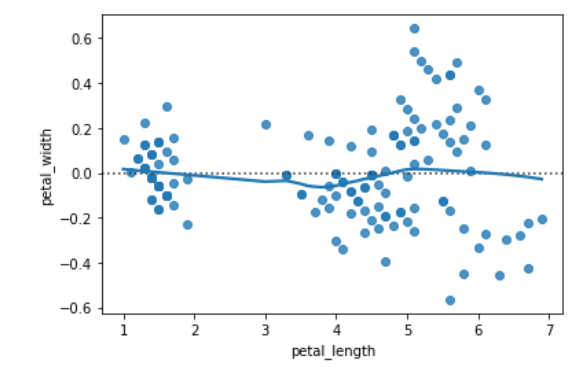Related Articles

# Python – seaborn.residplot() method

• Last Updated : 17 Aug, 2020

Seaborn is an amazing visualization library for statistical graphics plotting in Python. It provides beautiful default styles and color palettes to make statistical plots more attractive. It is built on the top of matplotlib library and also closely integrated to the data structures from pandas.

## seaborn.residplot() :

This method is used to plot the residuals of linear regression. This method will regress y on x and then draw a scatter plot of the residuals. You can optionally fit a lowess smoother to the residual plot, which can help in determining if there is a structure to the residuals.

Syntax: seaborn.residplot(x, y, data=None, lowess=False, x_partial=None, y_partial=None, order=1,
robust=False, dropna=True, label=None, color=None, scatter_kws=None, line_kws=None, ax=None)

Parameters: The description of some main parameters are given below:

• x: Data or column name in ‘data’ for the predictor variable.
• y: Data or column name in ‘data’ for the response variable.
• data: (optional) DataFrame having `x` and `y` are column names.
• lowess: (optional) Fit a lowess smoother to the residual scatterplot.
• dropna: (optional) This parameter takes boolean value. If True, ignore observations with missing data when fitting and plotting.

Return: Axes with the regression plot.

Below is the implementation of above method:

Example 1:

## Python3

 `# importing required packages``import` `seaborn as sns``import` `matplotlib.pyplot as plt`` ` `# loading dataset``data ``=` `sns.load_dataset(``"tips"``)`` ` `# draw residplot``sns.residplot(x ``=` `"total_bill"``,``              ``y ``=` `"tip"``,``              ``data ``=` `data)`` ` `# show the plot``plt.show()`` ` `# This code is contributed``# by Deepanshu Rustagi.`

Output:Example 2:

## Python3

 `# importing required packages``import` `seaborn as sns``import` `matplotlib.pyplot as plt`` ` `# loading dataset``data ``=` `sns.load_dataset(``"iris"``)`` ` `# draw residplot``# with lowess = True``sns.residplot(x ``=` `"petal_length"``,``              ``y ``=` `"petal_width"``,``              ``data ``=` `data,``              ``lowess ``=` `True``)`` ` `# show the plot``plt.show()`` ` `# This code is contributed``# by Deepanshu Rustagi.`

Output:Attention geek! Strengthen your foundations with the Python Programming Foundation Course and learn the basics.

To begin with, your interview preparations Enhance your Data Structures concepts with the Python DS Course. And to begin with your Machine Learning Journey, join the Machine Learning – Basic Level Course

My Personal Notes arrow_drop_up